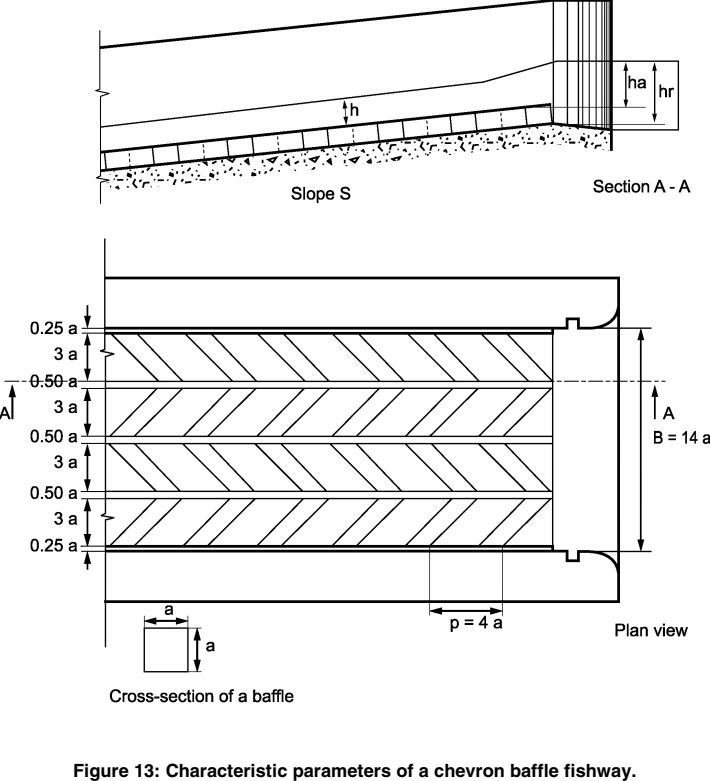# Mixed / chevron baffles fishwayExcerpt from Larinier, 20021

## Hydraulic laws given by abacuses

Experiments conducted by Larinier, 20021 allowed to establish abacuses that link adimensional flow $$q^*$$ :

$q^* = \dfrac{Q/L}{\sqrt{2g}a^{1,5}}$

to upstream head $$ha$$ and the average water level in the pass $$h$$ :Abacuses of a mixed / chevron baffles fishway for a slope of 10% (Excerpt from Larinier, 20021)Abacuses of a mixed / chevron baffles fishway for a slope of 15% (Excerpt from Larinier, 20021)

To run calculations for all slopes between 8% and 22%, polynomes coefficients of abacuses above are themelves adjusted in the form of slope $$S$$ depending polynomes.

We thus have:

$ha/a = a_2(S) q^{*2} + a_1(S) q^* + a_0(S)$
$a_2(S) = 0.188324S - 0.0427461$
$a_1(S) = - 2.47998S + 1.25363$
$a_0(S) = 5.02138S + 0.709434$

And:

$h/a = b_2(S) q^{*2} + b_1(S) q^* + b_0$
$b_2(S) = - 0.0733832S - 0.00839864$
$b_1(S) = 0.176261S + 0.661656$
$b_0(S) = - 4.97686S + 1.30546$

## Calculation of $$ha$$, $$h$$ and $$Q$$

We can then use those coefficients to calculate $$ha$$, $$h$$ and $$q^*$$:

$ha = a \left( a_2 (q^*)^2 + a_1 q^* + a_0 \right)$
$h = a \left( b_2 (q^*)^2 + b_1 q^* + b_0 \right)$

Using the positive inverse function, depending on $$ha/L$$, we get:

$q^* = \dfrac{-a_1 + \sqrt{a_1^2 - 4 a_2 (a_0 - h_a/a)}}{2 a_2}$

And we finally have:

$Q = L q^* \sqrt{g} a^{1,5}$

Calculation limitations of $$q^*$$, $$ha/a$$ and $$h/a$$ are determined based on the extremities of the abacuses curves.

## Flow velocity

Flow velocity $$V$$ corresponds to the minimum flow speed given the flow section $$A_w$$ at the perpendicular of the baffle :

$V = \dfrac{Q}{A_w}$

for mixed / chevron baffles fishways using the notation of the schema above, we have:

$A_w = h \times L$

## Upstream apron elevation $$Z_{r1}$$

$Z_{r1} = Z_{d1} + \frac{3 a S - a}{\sqrt{1 + S^2}}$

1. Larinier, M. 2002. “BAFFLE FISHWAYS.” Bulletin Français de La Pêche et de La Pisciculture, no. 364: 83–101. doi:10.1051/kmae/2002109